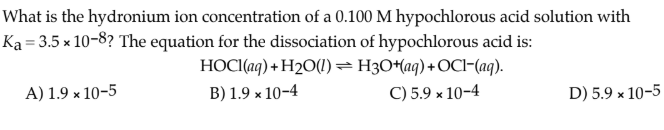# What is the hydronium ion concentration of a 0.100 M hypochlorous acid solution with Ka = 3.5 × 10^-8? The equation for the dissociation of hypochlorous acid is: HOCl(aq) + H2O(l) ⇌ H3O+(aq) +OCl-(aq). A) 1.9 × 10^-5 B) 1.9 × 10^-4 C) 5.9 × 10^-4 D) 5.9 × 10^-5